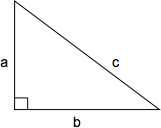## ASVAB Math Knowledge Practice Test 4007

Question 2 of 5
###### Pythagorean Theorem

The Pythagorean theorem defines the relationship between the side lengths of a right triangle. The length of the hypotenuse squared (c2) is equal to the sum of the two perpendicular sides squared (a2 + b2): c2 = a2 + b2 or, solved for c, $$c = \sqrt{a + b}$$If side a = 7, side b = 1, what is the length of the hypotenuse of this right triangle?

 $$\sqrt{10}$$ $$\sqrt{50}$$ $$\sqrt{65}$$ $$\sqrt{61}$$